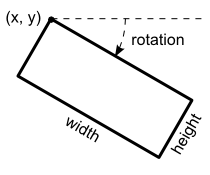2021.1.15

• java.lang.Object

• ```public class TLspLabelObstacle
extends java.lang.Object```
An obstacle: a location where label placement should be avoided. An obstacle can be anything, such as for example the objects that are labeled themselves, other important objects on the map that should not be obscured by labels, ...

An obstacle is a rotated rectangle expressed in view coordinates (pixels), see illustration.Obstacles are used by the `ILspLabelConflictChecker` interface and implementations.

Since:
2012.0
• ### Constructor Summary

Constructors
Constructor and Description
`TLspLabelObstacle()`
Constructs a new, uninitialized `TLspLabelObstacle`.
```TLspLabelObstacle(double aX, double aY, double aWidth, double aHeight, double aRotation)```
Constructs a new `TLspLabelObstacle` with the given parameters.
• ### Method Summary

All Methods
Modifier and Type Method and Description
`double` `getHeight()`
Returns the height.
`double` `getRotation()`
Returns the rotation of the obstacle in radians, clockwise, 0 radians at 3 o'clock.
`double` `getWidth()`
Returns the width.
`double` `getX()`
Returns x.
`double` `getY()`
Returns y.
`void` `setHeight(double aHeight)`
Sets the height of this obstacle, expressed in view coordinates (pixels).
`void` `setRotation(double aRotation)`
Sets the rotation of the obstacle in radians, clockwise, 0 radians at 3 o'clock.
`void` `setWidth(double aWidth)`
Sets the width of this obstacle, expressed in view coordinates (pixels).
`void` `setX(double aX)`
Sets the x-coordinate of the upper left corner of this obstacle, expressed in view coordinates (pixels).
`void` `setY(double aY)`
Sets the y-coordinate of the upper left corner of this obstacle, expressed in view coordinates (pixels).
• ### Methods inherited from class java.lang.Object

`clone, equals, finalize, getClass, hashCode, notify, notifyAll, toString, wait, wait, wait`
• ### Constructor Detail

• #### TLspLabelObstacle

`public TLspLabelObstacle()`
Constructs a new, uninitialized `TLspLabelObstacle`.
• ### Method Detail

• #### setX

`public void setX(double aX)`
Sets the x-coordinate of the upper left corner of this obstacle, expressed in view coordinates (pixels).
Parameters:
`aX` - The x.
• #### setY

`public void setY(double aY)`
Sets the y-coordinate of the upper left corner of this obstacle, expressed in view coordinates (pixels).
Parameters:
`aY` - The y.
• #### getWidth

`public double getWidth()`
Returns the width.
Returns:
the width.
`setWidth(double)`
• #### setWidth

`public void setWidth(double aWidth)`
Sets the width of this obstacle, expressed in view coordinates (pixels).
Parameters:
`aWidth` - The width.
• #### getHeight

`public double getHeight()`
Returns the height.
Returns:
the height.
`setHeight(double)`
• #### setHeight

`public void setHeight(double aHeight)`
Sets the height of this obstacle, expressed in view coordinates (pixels).
Parameters:
`aHeight` - The height.
• #### getRotation

`public double getRotation()`
Returns the rotation of the obstacle in radians, clockwise, 0 radians at 3 o'clock.
Returns:
the rotation of the obstacle.
`setRotation(double)`
`public void setRotation(double aRotation)`
`aRotation` - The rotation.